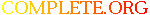Complete.Org: Mailing Lists: Archives: freeciv-ai: November 2002: [freeciv-ai] Re: Measure the AI ability to harvest resources# [freeciv-ai] Re: Measure the AI ability to harvest resources

[Top] [All Lists]

 To: freeciv-ai@xxxxxxxxxxx Subject: [freeciv-ai] Re: Measure the AI ability to harvest resources From: Raimar Falke Date: Mon, 25 Nov 2002 00:48:21 +0100

```On Sun, Nov 24, 2002 at 10:53:13PM +0100, Raimar Falke wrote:

I collected data what the players were building:

> all 4 player are controlled by server-AI:
>
> 2: 29:            Wang Gon:(f s t)=(  75  125  138) (l t s)=(   0   11  127)
> s=  5206 uf= 429
> 2: 29:      Guntis Ulmanis:(f s t)=( 113  147  146) (l t s)=(   0    1  145)
> s=  6398 uf= 440
> 2: 29:   Richard Lionheart:(f s t)=( 112  189   59) (l t s)=(   0   25   34)
> s=  6049 uf= 499
> 2: 29:             Genghis:(f s t)=( 105   60  144) (l t s)=(   0    0  144)
> s=  4743 uf= 408

2: 29:Wang Gon
2:               Warriors: 1 * 10 = 10
2:               Barracks: 2 * 40 = 80
2:   total = 90 shields
2: 29:Guntis Ulmanis
2:               Settlers: 1 * 40 = 40
2:               Barracks: 1 * 40 = 40
2:   total = 80 shields
2: 29:Richard Lionheart
2:               Settlers: 3 * 40 = 120
2:               Warriors: 1 * 10 = 10
2:               Barracks: 1 * 40 = 40
2:   total = 170 shields
2: 29:Genghis
2:               Settlers: 1 * 40 = 40
2:               Barracks: 1 * 40 = 40
2:   total = 80 shields

All have built Barracks. This is IMHO bad.

Wang Gon even built two Barracks and no Settler at all!!

> 2: 29:            Wang Gon:(f s t)=(  71  147  150) (l t s)=(   0   42  108)
> s=  5648 uf=4000
> 2: 29:      Guntis Ulmanis:(f s t)=( 123  160  162) (l t s)=(   0   11  151)
> s=  7001 uf=4000
> 2: 29:   Richard Lionheart:(f s t)=( 108  195   92) (l t s)=(   0   30   62)
> s=  6471 uf=4000
> 2: 29:             Genghis:(f s t)=( 145  173  139) (l t s)=(   0   19  120)
> s=  7364 uf=4000

2: 29:Wang Gon
2:               Settlers: 1 * 40 = 40
2:               Warriors: 1 * 10 = 10
2:               Barracks: 2 * 40 = 80
2:   total = 130 shields
2: 29:Guntis Ulmanis
2:               Settlers: 2 * 40 = 80
2:               Barracks: 1 * 40 = 40
2:   total = 120 shields
2: 29:Richard Lionheart
2:               Settlers: 3 * 40 = 120
2:               Warriors: 1 * 10 = 10
2:               Barracks: 1 * 40 = 40
2:   total = 170 shields
2: 29:Genghis
2:               Settlers: 3 * 40 = 120
2:               Barracks: 1 * 40 = 40
2:   total = 160 shields

They didn't build more barracks.

> agents control Genghis (now the highest player):
> 2: 29:            Wang Gon:(f s t)=(  71  147  150) (l t s)=(   0   42  108)
> s=  5648 uf=4000
> 2: 29:      Guntis Ulmanis:(f s t)=( 123  160  162) (l t s)=(   0   11  151)
> s=  7001 uf=4000
> 2: 29:   Richard Lionheart:(f s t)=( 108  195   92) (l t s)=(   0   30   62)
> s=  6471 uf=4000
> 2: 29:             Genghis:(f s t)=( 163  246  173) (l t s)=(   0   66  107)
> s=  9355 uf=4000
> +25%

2: 29:Genghis
2:                Siedler: 5 * 40 = 200
2:   total = 200 shields

> I haven't searched why the server AIs perform badly. It is quite
> possible that they built warriors or barracks.

Looks like I know the errors of the server-AI.

Raimar

--
email: rf13@xxxxxxxxxxxxxxxxx
"Are you saying that you actually used the Classpath Java AWT classes in
addition to the GTK peers and got them to display something?
Wow.  That's way better than I did and I wrote the code!"
-- Aaron M. Renn in the classpath mailing list

```